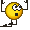# Speed of a Magnet Falling through a Conducting Wire

## Homework Statement

A small but very powerful bar magnet falls from rest under gravity through the center of a horizontal ring of conducting wire, as shown in the figure below (on the left). The speed-versus-time graph, in arbitrary units, of the magnet will correspond most closely to which of the following four plots below (on the right)?## Homework Equations

Lenz's law
$$\mathcal E=-{\frac {\partial {\Phi}_B}{\partial t}}$$

## The Attempt at a Solution

As the magnet falls towards the ring, the magnetic flux through the ring changes which induces an e.m.f. in the ring such that the motion of the magnet is opposed and its speed is reduced.
This continues until the magnet enters the ring when the flux is zero and consequently the speed reaches minimum value.

After the magnet has crossed the ring the flux through the ring again changes, generating an e.m.f. which opposes the motion of the magnet. But as the distance between the magnet and the ring increases this opposing force becomes weaker and the bar falls with almost constant acceleration giving a straight line, as i Fig. (a).

So the correct option is (a).

Is this correct?

#### Attachments

• 18.8 KB Views: 309

Related Introductory Physics Homework Help News on Phys.org
kuruman
Homework Helper
Gold Member
It looks right.

•VSayantan
It looks right.
Thanks @kurumanCWatters
Homework Helper
Gold Member
It's also quite easy to rule out the other options. In some the velocity falls to zero, in one it doesn't accelerate again after passing through the hoop.

•VSayantan
How convinced are we that the answer is a.?

The graph basically says the following:
• First linear slope: The speed of the bar magnet increases positively for a period of time
• Nearing the crest: The bar magnet starts slowing down in the positive direction
• Top of crest: The speed of the bar magnet is constant for a brief period of time
• Leaving the crest: The bar magnet starts speeding up in the negative direction
• Second linear slope: The speed of the bar magnet increases negatively for a period of time
• Nearing the trough: The bar magnet starts slowing down in the negative direction
• Bottom of trough: The speed of the bar magnet is constant for a brief period of time
• Leaving the trough: The bar magnet starts speeding up in the positive direction
• Third linear slope: The speed of the bar magnet increases positively for the remaining portion of time
The problem is that this answer choice suggests the bar magnet changes its direction of motion twice.

•VSayantan
CWatters
Homework Helper
Gold Member
No that's not a correct analysis of "a". Nowhere does it imply a change of direction. The speed never falls to zero.

•VSayantan
kuruman
Homework Helper
Gold Member
The problem is that this answer choice suggests the bar magnet changes its direction of motion twice.
Isn't it true that if the direction of motion (in one dimension) changes, the velocity goes through zero in which case the speed should also go through zero? There is no such time at which this happens in graph (a).

Also, it would be helpful if you were more precise in statements such as
The speed of the bar magnet increases negatively for a period of time
by stating the direction of the velocity and the direction of the acceleration that you think is the case.

•VSayantan
Isn't it true that if the direction of motion (in one dimension) changes, the velocity goes through zero in which case the speed should also go through zero? There is no such time at which this happens in graph (a).

Also, it would be helpful if you were more precise in statements such as

by stating the direction of the velocity and the direction of the acceleration that you think is the case.
You're correct; I misread it for some reason as a position versus time graph. As far as 'speed increasing negatively' is concerneed, that's a perfectly valid way of specifying velocity.

kuruman
Homework Helper
Gold Member
As far as 'speed increasing negatively' is concerneed, that's a perfectly valid way of specifying velocity.
I prefer to think that speed is increasing when the acceleration has a component in the direction of the velocity and decreasing when the acceleration has a component opposite to the velocity. Positive and negative are associated with arbitrary choices of reference frames.

•VSayantan
I prefer to think that speed is increasing when the acceleration has a component in the direction of the velocity and decreasing when the acceleration has a component opposite to the velocity. Positive and negative are associated with arbitrary choices of reference frames.
Indeed.

•VSayantan